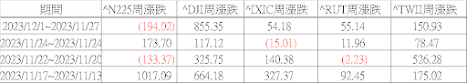## 2020年10月28日 星期三

### Excel VBA FOR 迴圈 簡單教學

同事卡在迴圈問題，按課本說得，可以作出99乘法表，但是確被書籍的課後練習題卡關了，所以寫在BLOG當作記錄跟分享。

CODE：

ActiveSheet.Cells.Clear

For I = 1 To 6 Step 1

ActiveSheet.Cells(I, 1) = 1   '

Next I

CODE：

ActiveSheet.Cells.Clear

For I = 1 To 6 Step 1

ActiveSheet.Cells(1, I) = 1

Next I

99乘法表：

Cells(J, I) 為 J 控制列，I 控制行

CODE：

ActiveSheet.Cells.Clear

For I = 1 To 9 Step 1

For J = 1 To 9 Step 1

ActiveSheet.Cells(J, I) = I * J

Next J
Next ICODE：

ActiveSheet.Cells.Clear

For I = 1 To 6 Step 1

For J = 1 To 6 Step 1

ActiveSheet.Cells(I, J) = J - (I - 1)

Next J

Next ICODE：

For I = 1 To 6 Step 1

For J = 1 To 6 - (I - 1) Step 1

ActiveSheet.Cells(I, J) = J

Next J

Next ICODE：

ActiveSheet.Cells.Clear

For I = 1 To 6 Step 1

For J = 1 To 6 Step 1

If J <= (6 - (I - 1)) Then

ActiveSheet.Cells(I, J) = J

End If

Next J

Next I

SETP_FINISH控制列，則換下一列故為SETP_FINISH+1

CODE：

ActiveSheet.Cells.Clear

SETP_FINISH = 1

For I = 1 To 6 Step 1

If SETP_FINISH > 6 Then

Exit For

End If

If I <= (6 - (SETP_FINISH - 1)) Then

ActiveSheet.Cells(SETP_FINISH, I) = I

End If

If I = 6 Then

SETP_FINISH = SETP_FINISH + 1

I = 0

End If
Next IGOOD。分享，程式可以解決數學問題，但別把程式當數學看，多一點想像力跟觀察，自然會走出一條路。

## 2020年10月27日 星期二

### VBA入門：樞紐分析 小工具(列資料隱藏、增加與取消小計)

Private Sub CommandButton1_Click()

For A = 1 To ActiveSheet.PivotTables.Count Step 1

Debug.Print ActiveSheet.PivotTables(A).Name

Next

End Sub

Sub DELETE_All_PTFieldsRow()

For Each pf In ActiveSheet.PivotTables("PivotTable1").RowFields

pf.Orientation = xlHidden

Next pf

End Sub

With ActiveSheet.PivotTables("PivotTable1").PivotFields(ROW_NAME)

.Orientation = xlRowField

End With

End Sub

Sub PivotTable_Subtotals_CANEL(TAG)

Set pt = ActiveSheet.PivotTables(1)

With pt

For P = LBound(TAG) To UBound(TAG, 2) Step 1

.PivotFields(TAG(1, P)).Subtotals(1) = True

.PivotFields(TAG(1, P)).Subtotals(1) = False

Next

End With

### 指數變化(2023.12.02)

指數變化(2023.12.02) 自己整理給自己勘，不作為投資理財參考 REVIEW上周紀錄：      本周很忙，略 本周指數：